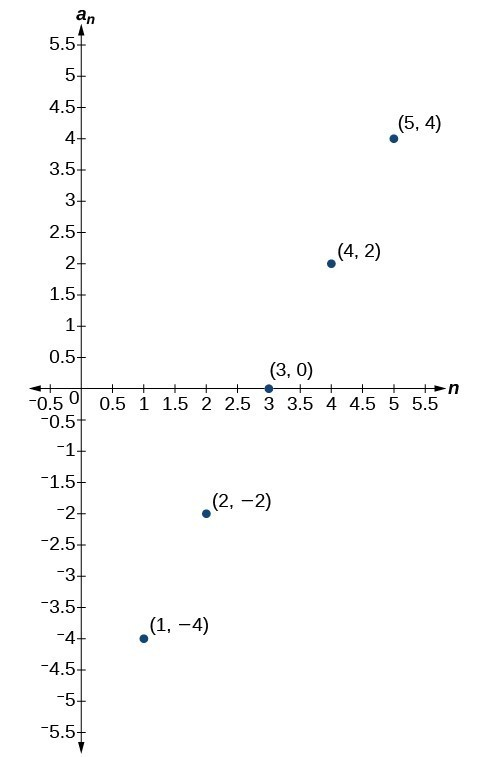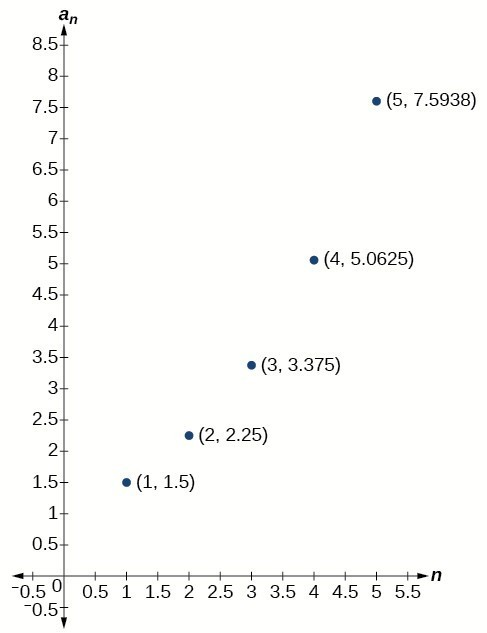## Section Exercises

1. What is an arithmetic sequence?

2. How is the common difference of an arithmetic sequence found?

3. How do we determine whether a sequence is arithmetic?

4. What are the main differences between using a recursive formula and using an explicit formula to describe an arithmetic sequence?

5. Describe how linear functions and arithmetic sequences are similar. How are they different?

For the following exercises, find the common difference for the arithmetic sequence provided.

6. $\left\{5,11,17,23,29,…\right\}$

7. $\left\{0,\frac{1}{2},1,\frac{3}{2},2,…\right\}$

For the following exercises, determine whether the sequence is arithmetic. If so find the common difference.

8. $\left\{11.4,9.3,7.2,5.1,3,…\right\}$

9. $\left\{4,16,64,256,1024,…\right\}$

For the following exercises, write the first five terms of the arithmetic sequence given the first term and common difference.

10. ${a}_{1}=-25$ , $d=-9$

11. ${a}_{1}=0$ , $d=\frac{2}{3}$

For the following exercises, write the first five terms of the arithmetic series given two terms.

12. ${a}_{1}=17,{a}_{7}=-31$

13. ${a}_{13}=-60,{a}_{33}=-160$

For the following exercises, find the specified term for the arithmetic sequence given the first term and common difference.

14. First term is 3, common difference is 4, find the 5th term.

15. First term is 4, common difference is 5, find the 4th term.

16. First term is 5, common difference is 6, find the 8th term.

17. First term is 6, common difference is 7, find the 6th term.

18. First term is 7, common difference is 8, find the 7th term.

For the following exercises, find the first term given two terms from an arithmetic sequence.

19. Find the first term or ${a}_{1}$ of an arithmetic sequence if ${a}_{6}=12$ and ${a}_{14}=28$.

20. Find the first term or ${a}_{1}$ of an arithmetic sequence if ${a}_{7}=21$ and ${a}_{15}=42$.

21. Find the first term or ${a}_{1}$ of an arithmetic sequence if ${a}_{8}=40$ and ${a}_{23}=115$.

22. Find the first term or ${a}_{1}$ of an arithmetic sequence if ${a}_{9}=54$ and ${a}_{17}=102$.

23. Find the first term or ${a}_{1}$ of an arithmetic sequence if ${a}_{11}=11$ and ${a}_{21}=16$.

For the following exercises, find the specified term given two terms from an arithmetic sequence.

24. ${a}_{1}=33$ and ${a}_{7}=-15$. Find ${a}_{4}$.

25. ${a}_{3}=-17.1$ and ${a}_{10}=-15.7$. Find ${a}_{21}$.

For the following exercises, use the recursive formula to write the first five terms of the arithmetic sequence.

26. ${a}_{1}=39;\text{ }{a}_{n}={a}_{n - 1}-3$

27. ${a}_{1}=-19;\text{ }{a}_{n}={a}_{n - 1}-1.4$

For the following exercises, write a recursive formula for each arithmetic sequence.

28. ${a}_{n}=\left\{40,60,80,…\right\}$

29. ${a}_{n}=\left\{17,26,35,…\right\}$

30. ${a}_{n}=\left\{-1,2,5,…\right\}$

31. ${a}_{n}=\left\{12,17,22,…\right\}$

32. ${a}_{n}=\left\{-15,-7,1,…\right\}$

33. ${a}_{n}=\left\{8.9,10.3,11.7,…\right\}$

34. ${a}_{n}=\left\{-0.52,-1.02,-1.52,…\right\}$

35. ${a}_{n}=\left\{\frac{1}{5},\frac{9}{20},\frac{7}{10},…\right\}$

36. ${a}_{n}=\left\{-\frac{1}{2},-\frac{5}{4},-2,…\right\}$

37. ${a}_{n}=\left\{\frac{1}{6},-\frac{11}{12},-2,…\right\}$

For the following exercises, write a recursive formula for the given arithmetic sequence, and then find the specified term.

38. ${a}_{n}=\left\{7\text{, }4\text{, }1\text{, }…\right\}$; Find the 17th term.

39. ${a}_{n}=\left\{4\text{, }11\text{, }18\text{, }…\right\}$; Find the 14th term.

40. ${a}_{n}=\left\{2\text{, }6\text{, }10\text{, }…\right\}$; Find the 12th term.

For the following exercises, use the explicit formula to write the first five terms of the arithmetic sequence.

41. ${a}_{n}=24 - 4n$

42. ${a}_{n}=\frac{1}{2}n-\frac{1}{2}$

For the following exercises, write an explicit formula for each arithmetic sequence.

43. ${a}_{n}=\left\{3,5,7,…\right\}$

44. ${a}_{n}=\left\{32,24,16,…\right\}$

45. ${a}_{n}=\left\{-5\text{, }95\text{, }195\text{, }…\right\}$

46. ${a}_{n}=\left\{-17\text{, }-217\text{, }-417\text{,}…\right\}$

47. ${a}_{n}=\left\{1.8\text{, }3.6\text{, }5.4\text{, }…\right\}$

48. ${a}_{n}=\left\{-18.1,-16.2,-14.3,…\right\}$

49. ${a}_{n}=\left\{15.8,18.5,21.2,…\right\}$

50. ${a}_{n}=\left\{\frac{1}{3},-\frac{4}{3},-3\text{, }…\right\}$

51. ${a}_{n}=\left\{0,\frac{1}{3},\frac{2}{3},…\right\}$

52. ${a}_{n}=\left\{-5,-\frac{10}{3},-\frac{5}{3},\dots \right\}$

For the following exercises, find the number of terms in the given finite arithmetic sequence.

53. ${a}_{n}=\left\{3\text{,}-4\text{,}-11\text{, }…\text{,}-60\right\}$

54. ${a}_{n}=\left\{1.2,1.4,1.6,…,3.8\right\}$

55. ${a}_{n}=\left\{\frac{1}{2},2,\frac{7}{2},…,8\right\}$

For the following exercises, determine whether the graph shown represents an arithmetic sequence.

56.57.For the following exercises, use the information provided to graph the first 5 terms of the arithmetic sequence.

58. ${a}_{1}=0,d=4$

59. ${a}_{1}=9;{a}_{n}={a}_{n - 1}-10$

60. ${a}_{n}=-12+5n$

For the following exercises, follow the steps to work with the arithmetic sequence ${a}_{n}=3n - 2$ using a graphing calculator:

• Press [MODE]
• Select SEQ in the fourth line
• Select DOT in the fifth line
• Press [ENTER]
• Press [Y=]
• $n\text{Min}$ is the first counting number for the sequence. Set $n\text{Min}=1$
• $u\left(n\right)$ is the pattern for the sequence. Set $u\left(n\right)=3n - 2$
• $u\left(n\text{Min}\right)$ is the first number in the sequence. Set $u\left(n\text{Min}\right)=1$
• Press [2ND] then [WINDOW] to go to TBLSET
• Set $\text{TblStart}=1$
• Set $\Delta \text{Tbl}=1$
• Set Indpnt: Auto and Depend: Auto
• Press [2ND] then [GRAPH] to go to the TABLE

61. What are the first seven terms shown in the column with the heading $u\left(n\right)\text{?}$

62. Use the scroll-down arrow to scroll to $n=50$. What value is given for $u\left(n\right)\text{?}$

63. Press [WINDOW]. Set $n\text{Min}=1,n\text{Max}=5,x\text{Min}=0,x\text{Max}=6,y\text{Min}=-1$, and $y\text{Max}=14$. Then press [GRAPH]. Graph the sequence as it appears on the graphing calculator.

For the following exercises, follow the steps given above to work with the arithmetic sequence ${a}_{n}=\frac{1}{2}n+5$ using a graphing calculator.

64. What are the first seven terms shown in the column with the heading $u\left(n\right)$ in the TABLE feature?

65. Graph the sequence as it appears on the graphing calculator. Be sure to adjust the WINDOW settings as needed.

66. Give two examples of arithmetic sequences whose 4th terms are $9$.

67. Give two examples of arithmetic sequences whose 10th terms are $206$.

68. Find the 5th term of the arithmetic sequence $\left\{9b,5b,b,\dots \right\}$.

69. Find the 11th term of the arithmetic sequence $\left\{3a - 2b,a+2b,-a+6b\dots \right\}$.

70. At which term does the sequence $\left\{5.4,14.5,23.6,…\right\}$ exceed 151?

71. At which term does the sequence $\left\{\frac{17}{3},\frac{31}{6},\frac{14}{3},…\right\}$ begin to have negative values?

72. For which terms does the finite arithmetic sequence $\left\{\frac{5}{2},\frac{19}{8},\frac{9}{4},…,\frac{1}{8}\right\}$ have integer values?

73. Write an arithmetic sequence using a recursive formula. Show the first 4 terms, and then find the 31st term.

74. Write an arithmetic sequence using an explicit formula. Show the first 4 terms, and then find the 28th term.